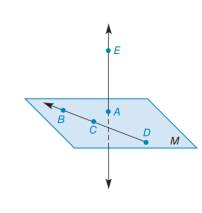Chapter 1.3, Problem 26E### Elementary Geometry for College St...

6th Edition
Daniel C. Alexander + 1 other
ISBN: 9781285195698

#### Solutions

Chapter
Section### Elementary Geometry for College St...

6th Edition
Daniel C. Alexander + 1 other
ISBN: 9781285195698
Textbook Problem
28 views

# In Exercises 9 to 28, use the drawings as needed to answer the following questions.Points A, B, C, and D are coplanar; B, C, and D are collinear; point E is not in plane M. How many planes containa) points A, B, and C?b) points B, C, and D?c) points A, B, C, and D?d) points A, B, C, and E?To determine

a)

To find:

Number of planes containing given points.

Explanation

Given:

The given figure is,

And, points A, B, C, and D are coplanar; B, C, and D are collinear; point E is not in plane M...

To determine

b)

To find:

Number of planes containing given points.

To determine

c)

To find:

Number of planes containing given points.

To determine

d)

To find:

Number of planes containing given points.

### Still sussing out bartleby?

Check out a sample textbook solution.

See a sample solution

#### The Solution to Your Study Problems

Bartleby provides explanations to thousands of textbook problems written by our experts, many with advanced degrees!

Get Started